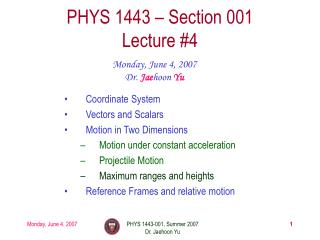DownloadDownload PresentationPHYS 1443 – Section 001 Lecture #4

# PHYS 1443 – Section 001 Lecture #4

Download Presentation## PHYS 1443 – Section 001 Lecture #4

- - - - - - - - - - - - - - - - - - - - - - - - - - - E N D - - - - - - - - - - - - - - - - - - - - - - - - - - -
##### Presentation Transcript

1. PHYS 1443 – Section 001Lecture #4 Monday, June 4, 2007 Dr. Jaehoon Yu • Coordinate System • Vectors and Scalars • Motion in Two Dimensions • Motion under constant acceleration • Projectile Motion • Maximum ranges and heights • Reference Frames and relative motion PHYS 1443-001, Summer 2007 Dr. Jaehoon Yu

2. Announcements • All of you but one have registered for the homework • Good job!!! • Mail distribution list • As of this morning there were 15 of you on the list • 7 more to go!! • Extra credit • 5 points if done by Today, June 4 • 3 points if done by Wednesday, June 6 PHYS 1443-001, Summer 2007 Dr. Jaehoon Yu

3. Displacement, Velocity and Speed Displacement Average velocity Average speed Instantaneous velocity Instantaneous speed PHYS 1443-001, Summer 2007 Dr. Jaehoon Yu

4. 1D Kinematic Equations of Motion on a Straight Line Under Constant Acceleration Velocity as a function of time Displacement as a function of velocities and time Displacement as a function of time, velocity, and acceleration Velocity as a function of Displacement and acceleration You may use different forms of Kinetic equations, depending on the information given to you for specific physical problems!! PHYS 1443-001, Summer 2007 Dr. Jaehoon Yu

5. +y y1 q1 +x x1 Coordinate Systems • They make it easy and consistent to express locations or positions • Two commonly used systems, depending on convenience, are • Cartesian (Rectangular) Coordinate System • Coordinates are expressed in (x,y) • Polar Coordinate System • Coordinates are expressed in distance from the origin ® and the angle measured from the x-axis, q (r,q) • Vectors become a lot easier to express and compute How are Cartesian and Polar coordinates related? (x1,y1) (r,q) = r1 O (0,0) PHYS 1443-001, Summer 2007 Dr. Jaehoon Yu

6. y q x qs (-3.50,-2.50)m Example Cartesian Coordinate of a point in the xy plane are (x,y)= (-3.50,-2.50)m. Find the equivalent polar coordinates of this point. r PHYS 1443-001, Summer 2007 Dr. Jaehoon Yu

7. Vector and Scalar Vector quantities have both magnitudes (sizes) and directions Force, gravitational acceleration, momentum Normally denoted in BOLD letters, F, or a letter with arrow on top Their sizes or magnitudes are denoted with normal letters, F, or absolute values: Scalar quantities have magnitudes only Can be completely specified with a value and its unit Energy, heat, mass, weight Normally denoted in normal letters, E Both have units!!! PHYS 1443-001, Summer 2007 Dr. Jaehoon Yu

8. y D F A B x E C Properties of Vectors sizes directions • Two vectors are the same if their and the are the same, no matter where they are on a coordinate system!! Which ones are the same vectors? A=B=E=D Why aren’t the others? C: The same magnitude but opposite direction: C=-A:A negative vector F: The same direction but different magnitude PHYS 1443-001, Summer 2007 Dr. Jaehoon Yu

9. A+B A+B A B A+B B B A A A-B A -B A B=2A Vector Operations • Addition: • Triangular Method: One can add vectors by connecting the head of one vector to the tail of the other (head-to-tail) • Parallelogram method: Connect the tails of the two vectors and extend • Addition is commutative: Changing order of operation does not affect the results A+B=B+A, A+B+C+D+E=E+C+A+B+D OR = • Subtraction: • The same as adding a negative vector:A - B = A + (-B) Since subtraction is the equivalent to adding a negative vector, subtraction is also commutative!!! • Multiplication by a scalar is increasing the magnitude A, B=2A PHYS 1443-001, Summer 2007 Dr. Jaehoon Yu

10. N Bsinq Bcosq E B 60o 20 q r A Example for Vector Addition A car travels 20.0km due north followed by 35.0km in a direction 60.0o west of north. Find the magnitude and direction of resultant displacement. Find other ways to solve this problem… PHYS 1443-001, Summer 2007 Dr. Jaehoon Yu

11. y Ay A q x Ax Components and Unit Vectors (+,+) }Components (Ax,Ay) Coordinate systems are useful in expressing vectors in their components (-,+) } Magnitude (-,-) (+,-) PHYS 1443-001, Summer 2007 Dr. Jaehoon Yu

12. Unit Vectors • Unit vectors are the ones that tells us the directions of the components • Dimensionless • Magnitudes are exactly 1 • Unit vectors are usually expressed in i, j, k or So the vector Acan be re-written as PHYS 1443-001, Summer 2007 Dr. Jaehoon Yu

13. Examples of Vector Operations Find the resultant vector which is the sum of A=(2.0i+2.0j) and B =(2.0i-4.0j) Find the resultant displacement of three consecutive displacements: d1=(15i+30j +12k)cm, d2=(23i+14j -5.0k)cm, and d3=(-13i+15j)cm Magnitude PHYS 1443-001, Summer 2007 Dr. Jaehoon Yu

14. Displacement, Velocity, and Acceleration in 2-dim • Average Velocity: • Displacement: How is each of these quantities defined in 1-D? • Instantaneous Velocity: • Average Acceleration • Instantaneous Acceleration: PHYS 1443-001, Summer 2007 Dr. Jaehoon Yu

15. Kinematic Quantities in 1D and 2D What is the difference between 1D and 2D quantities? PHYS 1443-001, Summer 2007 Dr. Jaehoon Yu